# Python+树莓派制作IoT（物联网）门控设备## 准备材料及工具

1.树莓派(Raspberry Pi 3B+) 数量：1块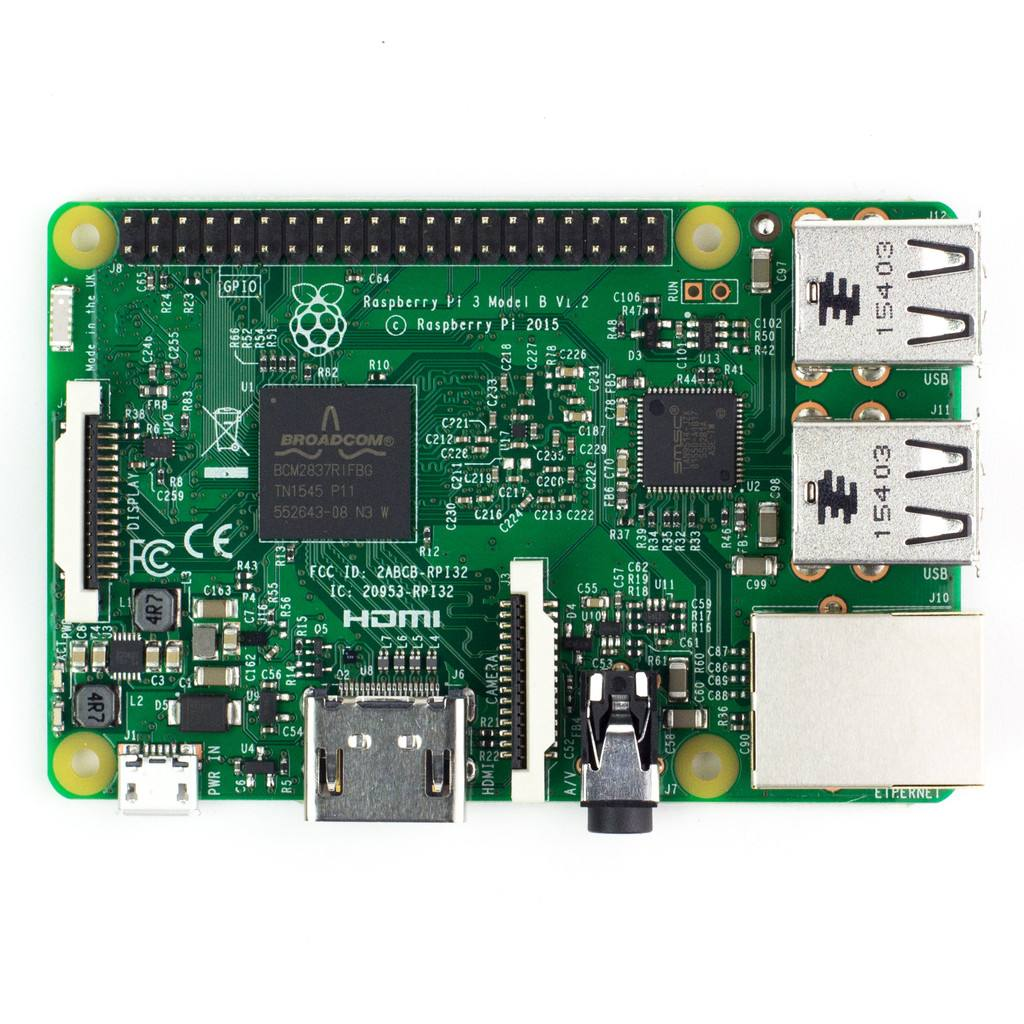2.SIM868通讯模块（这里使用的是适配树莓派的微雪电子的SIM868通讯模块） 数量：1块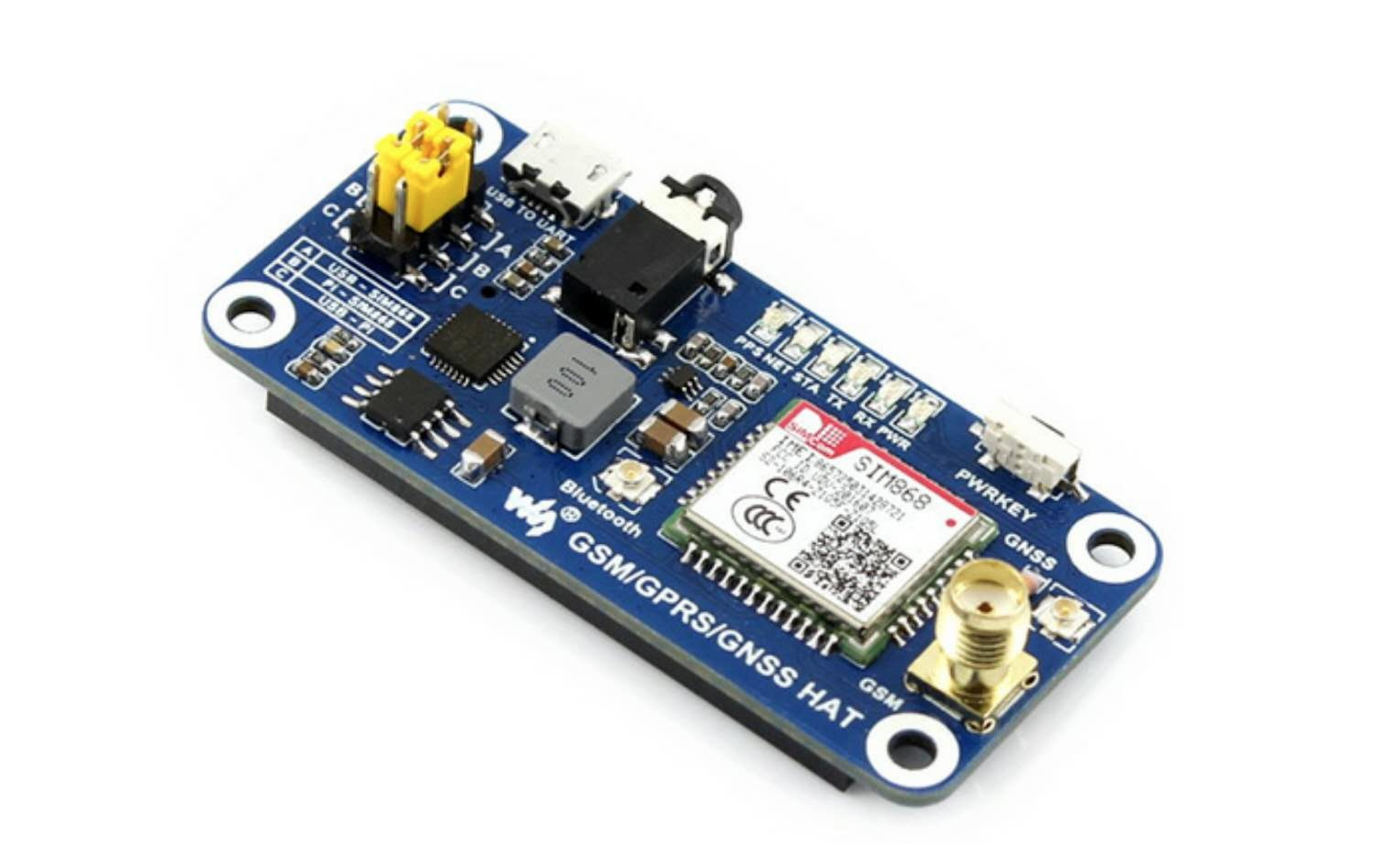3.电磁感应磁条（常开常闭型） 数量：1组

4.SIM868模块外接天线 数量：1根（根据现场安设实际情况确定）

5.树莓派T型扩展板 数量：1块

6.实验面包板 数量：1块

7.杜邦线（公对公，公对母） 数量：若干

8.太阳能板 数量：1块

9.带保护板的锂电池 数量：2组（根据电池使用的性能情况可适当增加）

10.电压电流转换板 数量：1块

11.连接电线 数量：（根据现场安设实际情况确定）

12.两芯屏蔽线 数量：10米（0.3粗即可根据现场安设实际情况确定）

13.防爆箱 （防尘、防雾） 数量：1个（规格根据实际情况确定）

14.Micro USB充电线 数量：2根

15.SIM卡（移动和联通均可，模块暂不支持电信CDMA） 数量：1张

16.电烙铁及焊锡

## 设备供电及模块链接说明

1. 供电原理：设备的供电依靠太阳能板配合锂电池进行供电，需要一块可以将太阳能板和锂电池的输出电压和电流转换成树莓派和SIM868通讯模块工作电压和电流的转换板，设备供电链接图如图。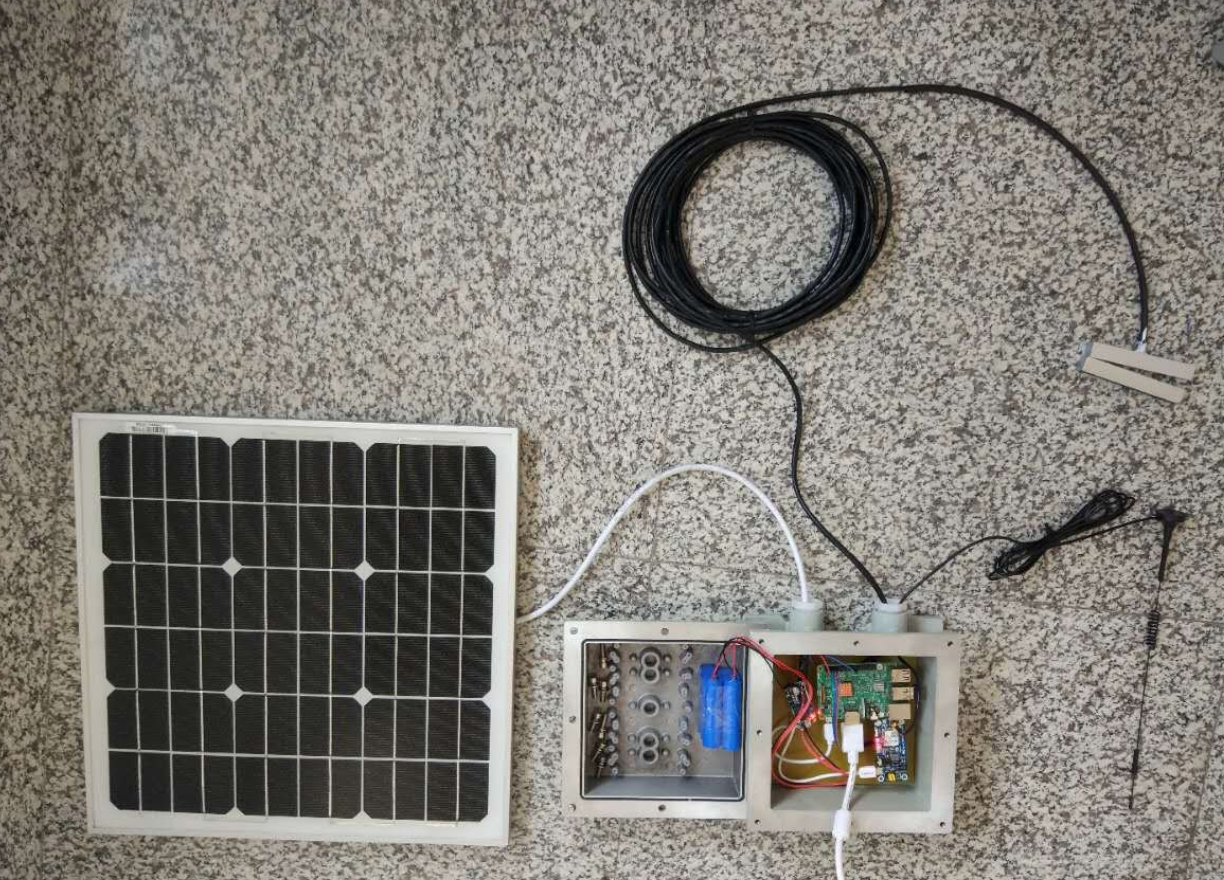实现效果，白天可以通过太阳能负责给树莓派及通讯模块供电并同时给锂电池充电。晚上将由充电完毕的锂电池负责给设备供电。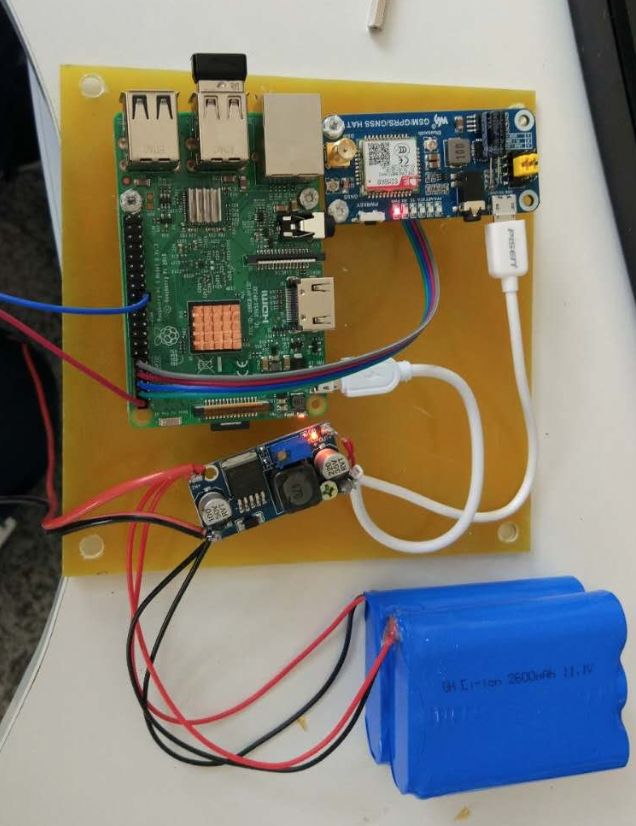2. 设备链接：树莓派的GPIO PIN# 2针脚（5V）和 PIN# 23针脚 链接电磁感应模块的引线。负责接收电磁感应模块的开关状态，树莓派的GPIO PIN# 4（5V），PIN# 6（Ground） ，PIN# 8（TX），PIN# 10（RX）分别链接SIM868通讯模块的5V，ground，串口TX和RX，负责实现模块的树莓派与SIM868通讯模块的串口通讯和供电链接，并将信号树莓派的接收到的电磁感应磁条开关信号，通过SIM868通讯模块出输出去（这里采用的是http传输协议，具体实现方法见程序编码部分）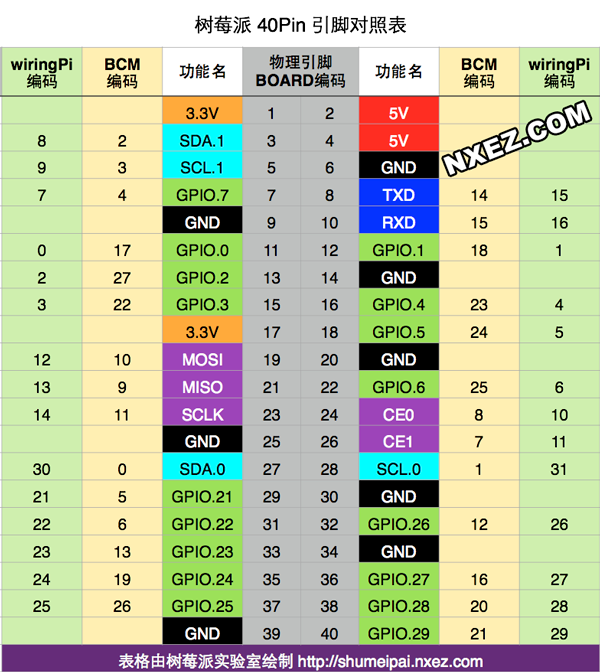## 程序代码

### python程序源码：

```import  RPi.GPIO as GPIO
import time
import serial

def gpio_init():
GPIO.setwarnings(False)
GPIO.setmode(GPIO.BOARD)
GPIO.setup(23,GPIO.OUT)
def send_data(param):
W_http_6='AT+HTTPPARA="URL","http://**************/iot.php?status='+param+'"\r\n'
ser.write(W_http_6)
time.sleep(2)
W_http_7='AT+HTTPACTION=0\r\n'
ser.write(W_http_7)
time.sleep(3)
if __name__ == '__main__':
ser = serial.Serial("/dev/ttyS0",115200)
W_http_1='AT+HTTPTERM\r\n'
ser.write(W_http_1)
time.sleep(3)
W_http_2='AT+SAPBR=3,1,"CONTYPE","GPRS"\r\n'
ser.write(W_http_2)
time.sleep(3)
W_http_3='AT+SAPBR=3,1,"APN","CMNET"\r\n'
ser.write(W_http_3)
time.sleep(3)
W_http_4='AT+SAPBR=1,1\r\n'
ser.write(W_http_4)
time.sleep(3)
W_http_5='AT+HTTPINIT\r\n'
ser.write(W_http_5)
time.sleep(3)
gpio_init()
status=1
while True:
if GPIO.input(23) == True:
if status==1:
send_data(2)
status=2
print("门的状态：关闭")
else:
pass
else:
if status==2:
send_data(1)
status=1
print("门的状态：打开")
else:
pass
time.sleep(3)
GPIO.cleanup()
```

### php程序源码

```require_once("../../func/dbaccess.php");
if (doConnect(\$cn) == false) {
return false;
}
\$strSQL = "insert into iot_tbl (position,status,create_time) values ("'.第一号门.'",'". \$_GET['status']."',now())";
doInsertUpdate(\$strSQL);
doClose(\$cn);
```

## 代码解析

```import  RPi.GPIO as GPIO
import time
import serial
```

```ser=serial.Serial("/dev/ttyS0",115200)
W_http_1='AT+HTTPTERM\r\n'
ser.write(W_http_1)
time.sleep(3)
W_http_2='AT+SAPBR=3,1,"CONTYPE","GPRS"\r\n'
ser.write(W_http_2)
time.sleep(3)
W_http_3='AT+SAPBR=3,1,"APN","CMNET"\r\n'ser.write(W_http_3)
time.sleep(3)
W_http_4='AT+SAPBR=1,1\r\n'ser.write(W_http_4)
time.sleep(3)
W_http_5='AT+HTTPINIT\r\n'ser.write(W_http_5)
time.sleep(3)
```

```def gpio_init():
GPIO.setwarnings(False)
GPIO.setmode(GPIO.BOARD)
GPIO.setup(23,GPIO.OUT)
```

gpio_init()函数是实现对接收电磁感应模块的GPIO PIN#23针脚的初始化配置。

```status=1
while True:
if GPIO.input(23) == True:
if status==1:
send_data(2)
status=2
print("门的状态：关闭")
else:
pass
else:
if status==2:
send_data(1)
status=1
print("门的状态：打开")
else:
pass
time.sleep(3)
```

```def send_data(param):
W_http_6='AT+HTTPPARA="URL","http://**************/iot.php?status='+param+'"\r\n'
ser.write(W_http_6)
time.sleep(2)
W_http_7='AT+HTTPACTION=0\r\n'
ser.write(W_http_7)
time.sleep(3)
```

```require_once("../../func/dbaccess.php");//封装链接操作MySQL数据库的函数
if (doConnect(\$cn) == false) {           //链接数据库
return false;
}
\$strSQL = "insert into iot_tbl (position,status,create_time) values ("'.第一号门.'",'".\$_GET['status']."',now())";  //将数据插入MySQL数据库的SQL语句
doInsertUpdate(\$strSQL);                 //执行SQL
doClose(\$cn);                            //关闭数据库链接
```